# MySQL令人咋舌的隐式转换

### 发布时间：2020-05-15 浏览次数：1068

`root@mysqldb 22:19:  [xucl]> select * from t1 where id=204027026112927603;+--------------------+| id                 |+--------------------+| 204027026112927605 || 204027026112927603 |+--------------------+2 rows in set (0.00 sec)`

`root@mysqldb 22:19:  [xucl]> select * from t1 where id=204027026112927603;+--------------------+| id                 |+--------------------+| 204027026112927605 || 204027026112927603 |+--------------------+2 rows in set (0.00 sec)`## 二、源码解释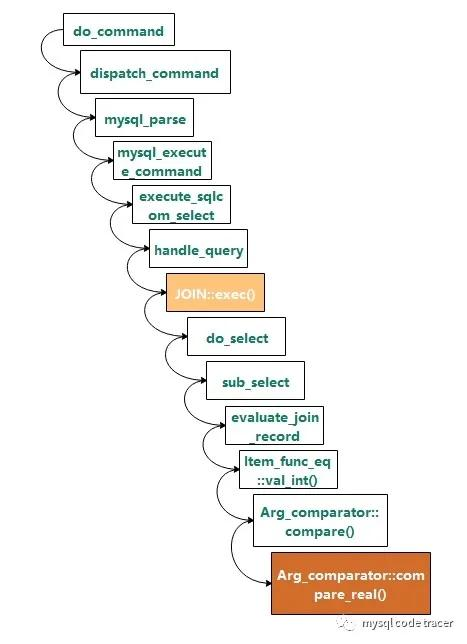`int Arg_comparator::compare_real(){  /*    Fix yet another manifestation of Bug#2338. 'Volatile' will instruct    gcc to flush double values out of 80-bit Intel FPU registers before    performing the comparison.  */  volatile double val1, val2;  val1= (*a)->val_real();  if (!(*a)->null_value)  {    val2= (*b)->val_real();    if (!(*b)->null_value)    {      if (set_null)        owner->null_value= 0;      if (val1 < val2)  return -1;      if (val1 == val2) return 0;      return 1;    }  }  if (set_null)    owner->null_value= 1;  return -1;}`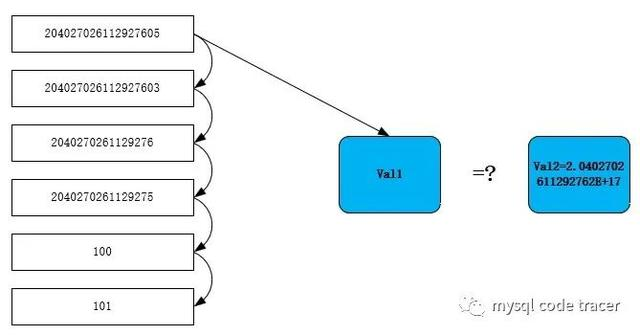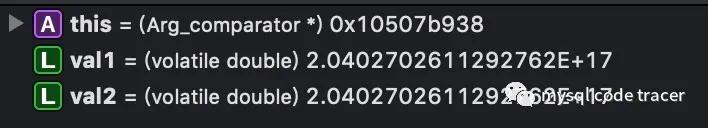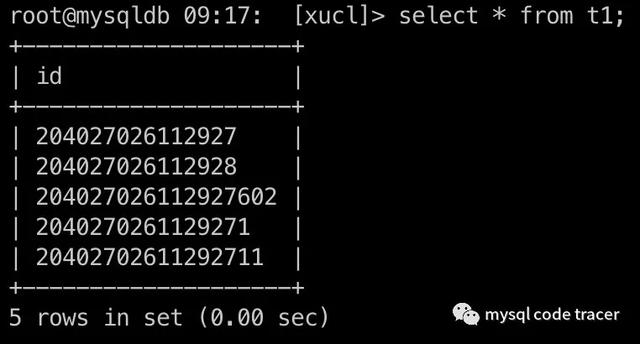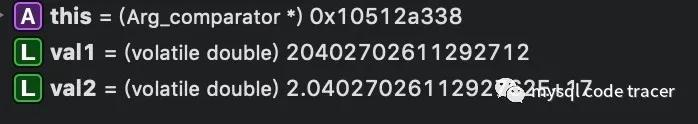#### MySQL string转成double的定义函数如下：

`root@mysqldb 22:19:  [xucl]> select * from t1 where id=204027026112927603;+--------------------+| id                 |+--------------------+| 204027026112927605 || 204027026112927603 |+--------------------+2 rows in set (0.00 sec)`

`/*  strtod for IEEE--arithmetic machines.   This strtod returns a nearest machine number to the input decimal  string (or sets errno to EOVERFLOW). Ties are broken by the IEEE round-even  rule.   Inspired loosely by William D. Clinger's paper "How to Read Floating  Point Numbers Accurately" [Proc. ACM SIGPLAN '90, pp. 92-101].   Modifications:    1. We only require IEEE (not IEEE double-extended).   2. We get by with floating-point arithmetic in a case that     Clinger missed -- when we're computing d * 10^n     for a small integer d and the integer n is not too     much larger than 22 (the maximum integer k for which     we can represent 10^k exactly), we may be able to     compute (d*10^k) * 10^(e-k) with just one roundoff.   3. Rather than a bit-at-a-time adjustment of the binary     result in the hard case, we use floating-point     arithmetic to determine the adjustment to within     one bit; only in really hard cases do we need to     compute a second residual.   4. Because of 3., we don't need a large table of powers of 10     for ten-to-e (just some small tables, e.g. of 10^k     for 0 <= k <= 22).*/`

`root@mysqldb 23:30:  [xucl]> select * from t1 where id=2040270261129276;+------------------+| id               |+------------------+| 2040270261129276 |+------------------+1 row in set (0.00 sec)root@mysqldb 23:30:  [xucl]> select * from t1 where id=101;+------+| id   |+------+| 101  |+------+1 row in set (0.00 sec)`

`root@mysqldb 22:19:  [xucl]> select * from t1 where id='204027026112927603';+--------------------+| id                 |+--------------------+| 204027026112927603 |+--------------------+1 row in set (0.01 sec)`

1. 避免发生隐式类型转换，隐式转换的类型主要有字段类型不一致、in参数包含多个类型、字符集类型或校对规则不一致等

2. 隐式类型转换可能导致无法使用索引、查询结果不准确等，因此在使用时必须仔细甄别

3. 数字类型的建议在字段定义时就定义为int或者bigint，表关联时关联字段必须保持类型、字符集、校对规则都一致

4. 最后贴一下官网对于隐式类型转换的说明

`1、If one or both arguments are NULL, the result of the comparison is NULL, except for the NULL-safe<=> equality comparison operator. For NULL <=> NULL, the result is true. No conversion is needed.2、If both arguments in a comparison operation are strings, they are compared as strings.3、If both arguments are integers, they are compared as integers.4、Hexadecimal values are treated as binary strings if not compared to a number.5、If one of the arguments is a TIMESTAMP or DATETIME column and the other argument is aconstant, the constant is converted to a timestamp before the comparison is performed. This isdone to be more ODBC-friendly. This is not done for the arguments to IN(). To be safe, alwaysuse complete datetime, date, or time strings when doing comparisons. For example, to achieve bestresults when using BETWEEN with date or time values, use CAST() to explicitly convert the values tothe desired data type.A single-row subquery from a table or tables is not considered a constant. For example, if a subqueryreturns an integer to be compared to a DATETIME value, the comparison is done as two integers.The integer is not converted to a temporal value. To compare the operands as DATETIME values,use CAST() to explicitly convert the subquery value to DATETIME. 6、If one of the arguments is a decimal value, comparison depends on the other argument. Thearguments are compared as decimal values if the other argument is a decimal or integer value, or asfloating-point values if the other argument is a floating-point value.7、In all other cases, the arguments are compared as floating-point (real) numbers.`